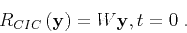Micro-earthquake monitoring with sparsely-sampled dataNext: Interferometric imaging condition Up: Micro-earthquake monitoring with sparsely-sampled Previous: Introduction

# Conventional imaging condition

Assuming dataacquired at coordinatesfunction of time(e.g. in a borehole) we can reconstruct the wavefieldat coordinatesin the imaging volume using an appropriate Green's functioncorresponding to the locationsand(Figure 2)(1)

where the symbolindicates time convolution. The total wavefieldat coordinatesdue to data recorded at all receivers located at coordinatesis represented by the superposition of the reconstructed wavefields:(2)

A conventional imaging condition (CIC) applied to this reconstructed wavefield extracts the imageas the wavefield at time(3)

This imaging procedure succeeds if several assumptions are fulfilled: first, the velocity model used for imaging has to be accurate; second, the numeric solution to the wave-equation used for wavefield reconstruction has to be accurate; third, the data need to be sampled densely and uniformly on the acquisition surface. In this paper, I assume that the first and third assumptions are not fulfilled. In these cases, the imaging is not accurate because contributions to the reconstructed wavefield from the receiver coordinates do not interfere constructively, thus leading to imaging artifacts. As indicated earlier, this situation is analogous to the case of imaging with an inaccurate velocity model, e.g. imaging with a smooth velocity of data corresponding to geology characterized by rapid velocity variations.

Different image processing procedures can be employed to reduce the random wavefield fluctuations. The procedure advocated in this paper uses interferometry for noise cancellation. Interferometric procedures can be formulated in various frameworks, e.g. coherent interferometric imaging (Borcea et al., 2006) or wave-equation migration with an interferometric imaging condition (Sava and Poliannikov, 2008).

coord
Figure 2.
Illustration of the variablesandused for the description of the conventional and interferometric imaging procedures.Micro-earthquake monitoring with sparsely-sampled dataNext: Interferometric imaging condition Up: Micro-earthquake monitoring with sparsely-sampled Previous: Introduction

2013-08-29